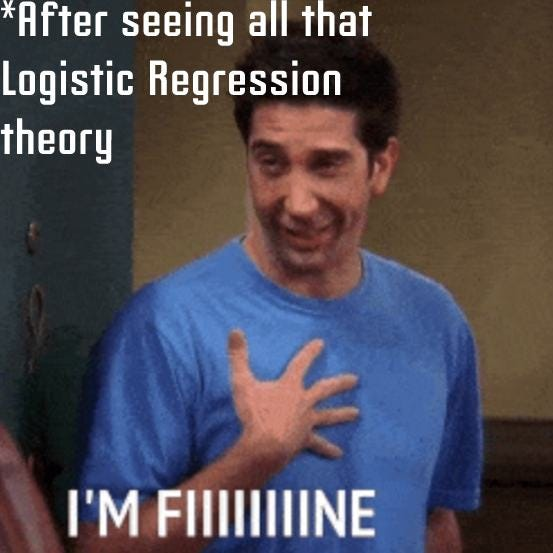You are more powerful than you know

# Naïve Bayes Classifier

Probabilistic machine learning model

A Naive Bayes classifier belongs to family of probabilistic machine learning model which is used for classification task. The groundwork of this classifier is based on the Bayes theorem.

So lets see what is Bayes Theorem

# Bayes Theorem

Following formula depicts Bayes Theorem where we find probability of A given B has occurred.

# Support Vector Machines: SVM

Complete guide from scratch

It is better if you first read about Linear Regression and Logistic Regression. If not please go and explore them (You can refer to following links for help).

Support Vector Machines is a very powerful classifier which can work both on linearly and non-linearly separable data. It can be used for regression as well as classification problems, yet primarily used for Classification problems.

There are many advantages associated to SVMs:

• Effective in high dimensional spaces.
• Still effective in cases where number of dimensions is greater than the number of samples.
• Uses a subset of training points…

# Image Segmentation Using K-Means

classification of an image into different groups

Image segmentation is a process of partitioning an image into multiple segments. The main motive behind this is to change the representation of image to more informative or we can focus on only those regions which are important.

## Applications

It is used in medical imaging, automatic cars, object detection, video surveillance and many more.

Prerequisite is to learn K-Means Algorithm

Lets see first detect most dominating colours :

`img = cv2.imread(‘./elephant.jpg’)plt.imshow(im)`

# K-means Clustering

From scratch explanation and implementation

K-means Clustering is an unsupervised machine learning technique. It aims to partition n observations into k clusters.

Kmeans algorithm is an iterative algorithm that tries to partition the dataset into K distinct non-overlapping subgroups (clusters) where each data point belongs to only one group

# Logistic Regression : All You wanna Know

Complete implementation from scratchLogistic Regression is used to model probability of a certain class so that it can be assigned value of 0 or 1. It is a statistical and supervised learning model. It is a go-to method for binary classification.

For instance you want to predict whether is person is having diabetes or not ! OR Let’s say you want to know the survival rate from any dataset OR Any mail is a spam (1) or not (0).

So basically it is a classification technique. Here we will see Binary Classification.

We use Sigmoid Function in Logistic Regression…

# Decision Tree & Random Forests

Complete Implementation From Scratch

Decision Tree is the most powerful and popular tool used for both classification and regression. It is like a flow-chart or we can a tree like model where every node depicts a feature while top node is called root node.

# k-nearest neighbours

MACHINE LEARNING ALGORITHM

KNN is a simple and intuitive Machine Learning Algorithm. It can be used for both classification and regression. It is a sort of Supervised Learning where we get both x and y.

KNN is Non-Parametric which means it makes no assumptions so accuracy depends on the quality of the data. It is easy to use as well as has a quick calculation time.

# Linear Regression

Single And Multiple Dependent Variables

Regression basically means when you want to predict a continuous data. Regression Analysis is a form of predictive modelling technique which investigates relation between dependent and independent variable.

# Probability & Statistics : Bed Rock Of Machine Learning

Machine Learning is an interdisciplinary field that uses statistics, probability, algorithms to learn from data and provide insights which can be used to build intelligent applications

## Joint Probability Distribution

Probability of events A and B denoted by`P(A and B) or P(A ∩ B)`is the probability that events A and B both occur. `P(A ∩ B) = P(A). P(B)` . This only applies if `A`and `B`are independent, which means that if `A`occurred, that doesn’t change the probability of `B`, and vice versa.

Prob(X=x, Y=y)

“Probability of X=x and Y=y”

p(x, y)

## Conditional Probability Distribution

Let us consider A and B are not independent, because if A occurred…

# Statistics In Machine Learning

Using Numpy and Statistics Module

Statistics is a collection of tools that you can use to get answers to important questions about data. We need statistics to help transform observations into information and to answer questions about samples of observations.

To summarize →

Statistics is a collection of tools developed over hundreds of years for summarizing data and quantifying properties of a domain given a sample of observations.